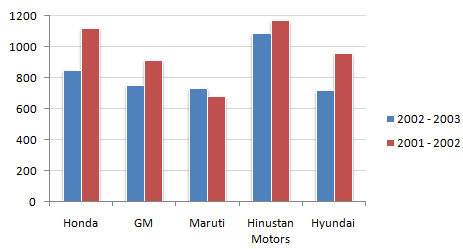# Data Interpretation - Bar Charts - Discussion

Study the following bar chart and answer the questions carefully.

Sales Turnover of 5 Companies (in Rs. crores)3.

Which of the companies shows the maximum percentage difference in sales turnover between the two years ?

 [A]. Honda [B]. GM [C]. Hyundai [D]. Maruti

Explanation:

Hyundai with 25.25 % is marginally higher than Honda with 24.5 %.

 Swojeena Shrestha said: (May 1, 2014) How can it be, there is no any real value in number to calculate to specify with the percentage you suggest?

 Praveen Kumar Kv said: (Jun 26, 2014) @Swojeena in question they ask Max percent diff. Going to answer there is only two companies near max Hyundai (250/700)*100% and Honda (250/850)*100%. Actually in fig we can't get exact values. as mine I take 50,100,150..etc..

 Kasinath @Hyd said: (Aug 20, 2014) Honda: (1100-850)/1100*100 = 22.72. Here we used divided by 1100 is just because the sales turnover is REDUCED from 2001-02 to 2002-03. In case of Increase we divide by 850. GM: (900-750)/900*100 = 16.6. Maruthi: (700-650)/650*100 = 7.69. We used divided by 650 is just because the sales turnover is INCREASED from 2001-02 to 2002-03. HM: (1150-1100)/1150*100 = 4.34. Hyundai: (950-700)/950*100 = 26.31 (MAX % Difference).

 Xyz said: (Apr 12, 2017) For Honda and Hyundai difference is nearly same. But for Hyundai base is less than Honda. Means less denominator and is larger. That's it.